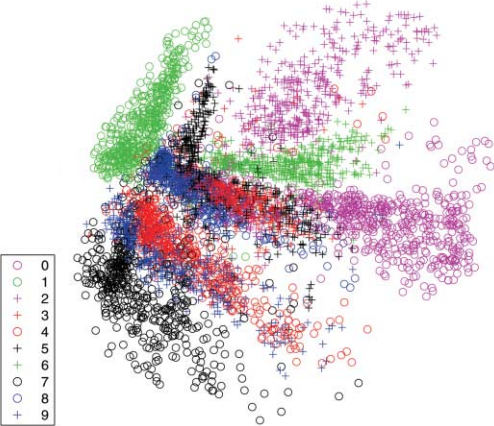# Generative Models

• Typical scenario: a model finds a representation $h = f(x)$ of a datapoint (image) $x$
• Generative models can produce an image $x$ from its representation $h$

## Autoencoders

• An encoder network followed by a decoder network
• Encoder compresses the data into a lower-dimensional vector
• Given powerful enough decoder, in theory the original datapoint could be perfectly reconstructed even from a one-dimensional latent representation
• A standard autoencoder learns representations with distinct clusters and lack of regularity in the latent space
• In order to generate new content we would need a way to sample meaningful latent representations

### Variational Autoencoder (VAE)

• VAE encoder produces two vectors: means and variances for a set of random variables
• The input of the decoder is a sample of these random variables
• The latent space is continuousTraining an autoencoder on MNIST results in distinct clusters. Hinton and Salakhutdinov.

• Simultaneously train two models, generator $G$ and discriminator $D$
• $G$ learns a mapping from a prior noise distribution to the data space
• $D$ predicts the probability that a sample is from the training data rather than was generated by $G$
• A conditional GAN is obtained by adding an additional input to both $G$ and $D$ (for example, image category or an input image)

### pix2pix

• The difficulty with training an image-to-image network is what loss to optimize
• For example, Euclidean distance is minimized by averaging all plausible outputs, producing blurry results
• GAN learns the loss function automatically
• A conditional GAN that is conditioned on the input image is suitable for image-to-image translation
• Generator is based on the U-Net architecture
• PatchGAN discriminator penalizes structure at the scale of local image patches only
• The discriminator is run convolutionally across the image, averaging responses from all patches
• Additional L1 loss encourages the output to be similar to the ground truth

### CycleGAN

• Two generators, $G$ and $F$, translate images between two domains
\begin{align} &G: X \to Y \\ &F: Y \to X \end{align}
• Generator is a CNN consisting of an encoder, transformer, and a decoder
• Two discriminators, $D_Y$ and $D_X$, try to distinguish real images from generated images
• Discriminator is a CNN that follows the PatchGAN architecture
• Adversarial loss for $G$ makes $D_Y$ distinguish $G(x)$ from $y$:
\begin{align} &D_Y(y) \to 0 \\ &D_Y(G(x)) \to 1 \end{align}
• Adversarial loss for $F$ makes $D_X$ distinguish $F(y)$ from $x$:
\begin{align} &D_X(x) \to 0 \\ &D_X(F(y)) \to 1 \end{align}
• Cycle consistency loss expresses that an image translation cycle should bring back the original image:
\begin{align} &F(G(x)) \to x \\ &G(F(y)) \to y \end{align}

## Reversible Generative Models

• GANs cannot encode images into the latent space
• VAEs support only approximate inference of latent variables from an image
• Normalizing flow is a sequence of invertible transformations
• Reversible generative models can encode an image into a latent space, making it possible to interpolate between two images